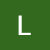# Basic Statistics for Data Science

Statistical analysis allows us to derive valuable insights from the data at hand. A sound grasp of the important statistical concepts and techniques is absolutely essential to analyze the data using various tools. This article deals with some fundamental statistical concepts that every data science enthusiast must know. Code snippets in python have also been included, wherever required, for better understanding.

Before we go into the details, let’s take a look at the topics covered in this article:

• Descriptive vs. Inferential Statistics
• Data Types
• Probability & Bayes’ Theorem
• Measures of Central Tendency
• Skewness
• Kurtosis
• Measures of Dispersion
• Covariance
• Correlation
• Probability…

# Introduction to Bayesian Statistics for Data Science and Analytics (Part -1)

Over the years, the data science domain has assumed great significance with the exponential growth of data. A solid foundation in the underlying mathematical concepts and statistics is vital to master data science and analytics. Bayesian statistics is a must-know for all data science and analytics professionals since data science has deep roots in the Bayesian approach.

In this article, we will look into:

1) What is Bayesian Statistics?

2) Bayesian statistics vs. Frequentist (Classical) statistics

3) Bayes’ theorem

The next article (Part-2) will deal with Bayesian inference and the diverse applications of Bayesian statistics in data science and analytics.## Lekshmi S. Sunil

Sophomore at Indian Institute of Technology (IIT) Indore